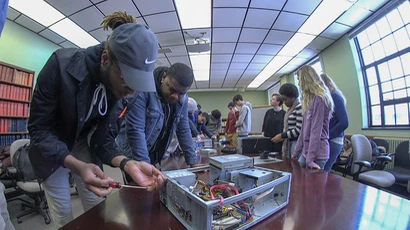# Grammars

The project was divided into two key parts. First being the generation of a string from a given grammar. The second part was taking the constructed string and drawing the graphic. This allows you the development of complex drawings by simply repeating a simple grammar rule.

String Generation: Each grammar contains an axiom or start symbol. When generating the string for each grammar this the process begins here.

Here is an example grammar:

F -> FF+F+F+F+FF
axiom F+F+F+F
TurtleDir 4

If chosen to generate a string with one cycle it will start with the axiom F+F+F+F, and then also generate the rule for F a single time for each F in the axiom. This results in a string of

FF+F+F+F+FF+FF+F+F+F+FF+FF+F+F+F+FF+FF+F+F+F+FF.

Now if this were to be run two cycles, then for every F in this string the rule for F will be implemented, generating a much longer string of

FF+F+F+F+FFFF+F+F+F+FF+FF+F+F+F+FF+FF+F+F+F+FF+FF+F+F+F+FF+FF+F+F+F+FFFF+F+F+F+FF+FF+F+F+F+FFFF+F+F+F+FF+FF+F+F+F+FF+FF+F+F+F+FF+FF+F+F+F+FF+FF+F+F+F+FFFF+F+F+F+FF+FF+F+F+F+FFFF+F+F+F+FF+FF+F+F+F+FF+FF+F+F+F+FF+FF+F+F+F+FF+FF+F+F+F+FFFF+F+F+F+FF+FF+F+F+F+FFFF+F+F+F+FF+FF+F+F+F+FF+FF+F+F+F+FF+FF+F+F+F+FF+FF+F+F+F+FFFF+F+F+F+FF.

It is quite easy to see how simple rules such as in this grammar can quietly lead to large strings. If ran another cycle there would be yet another rule of F for every F in the last string generation.

Grammars in essence can contain any number of rules. Take this next grammar for instance:

X -> X+YF+
Y -> -FX-Y
axiom X
TurtleDir 4

A single generation results in X+YF+.
A second generation results in a continuation using both rules X+YF++-FX-YF+, and so on.

Fractal drawing: These strings after being generated are then drawn using turtle graphics. The string generated is viewed as a list of commands for the turtle graphics. The character F draws a line and moves the turtle forward. The length of the line is determined by the size of the string, longer strings result in shorter lines. Any other grammar rule such as X,V,or O for example has no bearing on the turtle graphics and is ignored. Operations such as + and – change the direction in which the turtle is facing.

Here are a few screenshots from two of the grammars...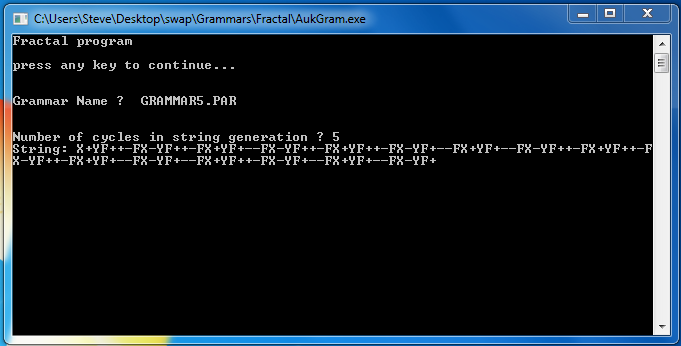This is a screenshot of the string generation of grammar 5.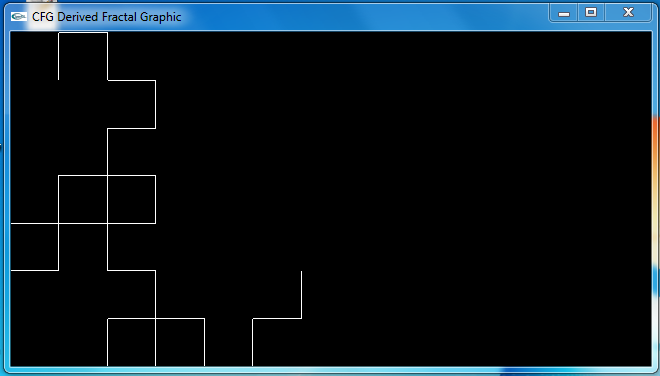This is a screenshot of the drawing of grammar 5.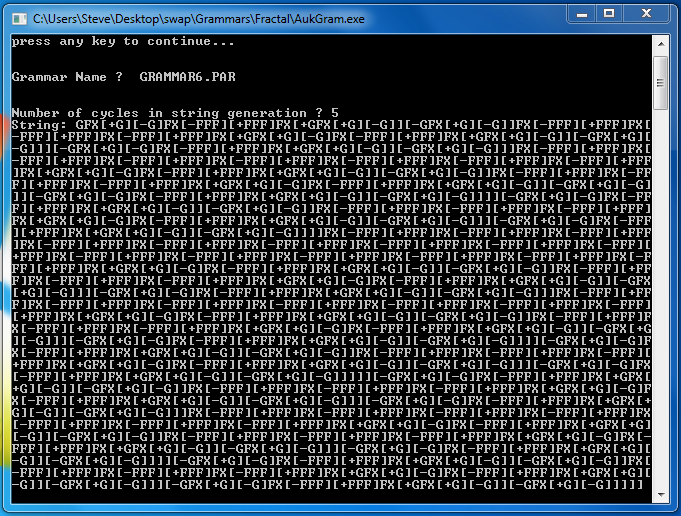This is a screenshot of the string generation of grammar 6.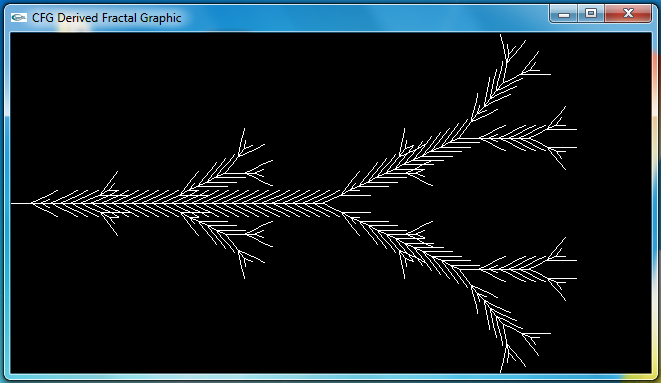This is a screenshot of the drawing of grammar 6.

This project was completed by Shawn Aukstakalnis for CSIT 499 Senior Project.